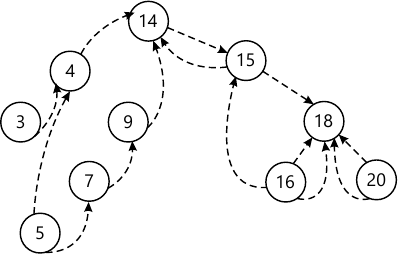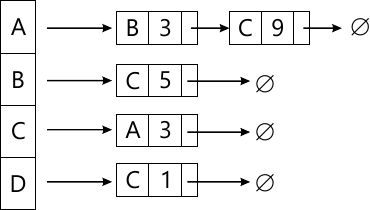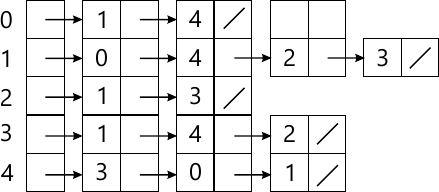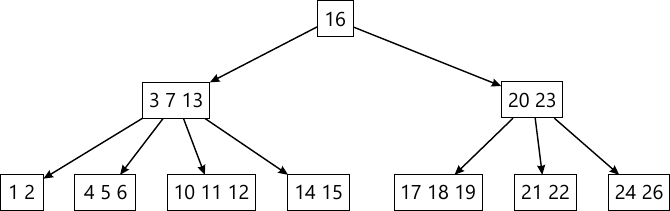MORE IN Data Structures Algorithm
SPPU Computer Engineering (Semester 3)
Data Structures Algorithm
December 2014
Total marks: --
Total time: --
INSTRUCTIONS
(1) Assume appropriate data and state your reasons
(2) Marks are given to the right of every question
(3) Draw neat diagrams wherever necessary

Answer any one question from Q1 and Q2
1 (a) Construct a logical expression for allowing purchasing using credit card if the following rules are satisfied. The card may be used if the
(i) Balance plus sales amount is less than the maximum allowable amount
(ii) Last payment was less than 45 days ago
(iii) Credit card has not expired.
3 M
1 (b) Sort the following data using Quick Sort Algorithm. 65, 70, 75, 80, 85, 60, 55, 50, 45. Show the output after every pass.
4 M
1 (c) Draw the threaded binary tree equivalent for the tree represented by the following array assuming root node of tree starts with index 1 in array.
 0 1 2 3 4 5 6 7 8 9 10 11
 10 - 20 - - 30 - - - - -
 12 13 14 15 16 17 18 19 20 21 22 23
 - 40 - - - - - - - - - -
 24 25 26 27 28 29 30 31
 - - 50 - - - - -
5 M

2 (a) Find the frequency count of the following code:
sum = 0;
for(i = 0; i < = n; i++)
for(j = 0; j < = n; j++)
sum = sum + i + j.
3 M
2 (b) Consider the following threaded binary search tree. Delete the root node of the tree and redraw the tree again with threading by maintaining the property of binary search Tree.4 M
2 (c) Write a non-recursive pseudo C/C++ code for any DFS traversal of binary tree.
5 M

Answer any one question from Q3 and Q4
3 (a) Consider the following graph represented using Adjacency List. Find the minimum spanning tree for the above graph by using Prim's Algorithm.4 M
3 (b) Write a pseudo C/C++ code for LR and RL rotations in AVL Trees.
6 M
3 (c) Enlist various collision resolution techniques.
2 M

4 (a) Draw the BFS traversal of the following graph represented using adjacency list4 M
4 (b) Construct the AVL tree for the following data by inserting each of the following data item one at a time: 10, 20, 15, 12, 25, 30, 14, 22, 35, 40.
5 M
4 (c) Enlist hash functions to calculate the hash values of the data.
3 M

Answer any one question from Q5 and Q6
5 (a) Consider the following 5 Way B Tree. Delete root node i.e. a node with key value 16 from the above tree and redraw the tree by maintaining its B Tree property.6 M
5 (b) Build the Min-Heap for the following data:
25, 12, 27, 30, 5, 10, 17, 29, 40, 35
4 M
5 (c) Explain any three operations performed on sequential files.
3 M

6 (a) Write a pseudo C/C++ code to sort the data using heap sort in ascending order.
7 M
6 (b) Create a 3 way B tree by inserting the following data one at a time:
5, 3, 21, 9, 1, 13, 2, 7, 10, 12, 4, 8
6 M

Answer any one question from Q7 and Q8
7 (a) Explain how parallel prefix computation algorithm can be applied to the following example: 5, 3, -6, 2, 7, 10, -2, 8. Assume the prefix operation as addition.
6 M
7 (b) Write a parallel algorithm for odd-even merge sort. Explain the algorithm with suitable example.
7 M

8 (a) Explain parallel pointer doubling algorithm with suitable example.
7 M
8 (b) Write a parallel algorithm to perform the addition of the given numbers using complete binary tree method.
6 M

More question papers from Data Structures Algorithm